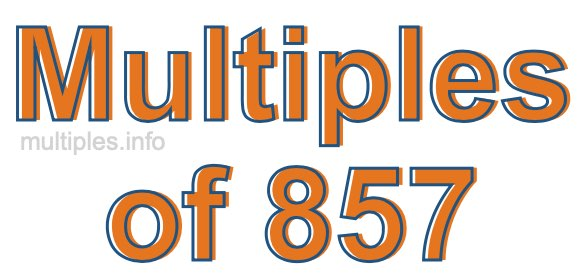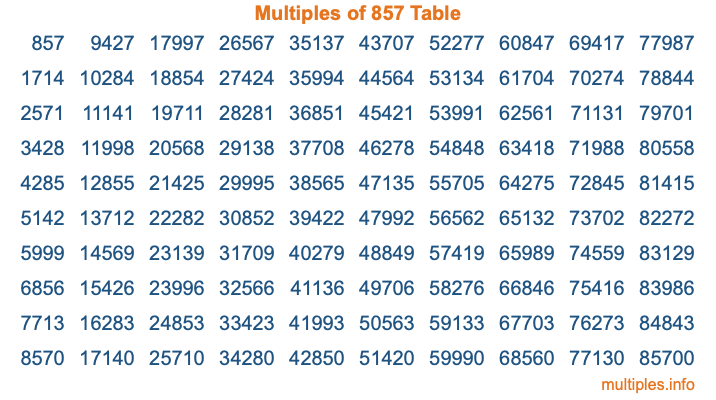Multiples of 857Welcome to the Multiples of 857 page. Here we will first teach you everything you will ever need to know about the multiples of 857, and then give you a study guide summary of everything we taught you to make sure you remember it all. Use this page to look up facts and learn information about the multiples of 857. This page will make you a multiples of eight hundred fifty-seven expert!

Definition of Multiples of 857
Multiples of 857 are all the numbers that when divided by 857 equal an integer. Each of the multiples of 857 are called a multiple. A multiple of 857 is created by multiplying 857 by an integer.

Therefore, to create a list of multiples of 857, you start with 1 multiplied by 857, then 2 multiplied by 857, then 3 multiplied by 857, and so on for as long as you want. Thus, the list of the first five multiples of 857 is 857, 1714, 2571, 3428, and 4285. To see a larger list of multiples of 857, see the printable image of Multiples of 857 further down on this page. We also have a category where you can choose any nth multiple of 857.

Multiples of 857 Checker
The Multiples of 857 Checker below checks to see if any number of your choice is a multiple of 857. In other words, it checks to see if there is any number (integer) that when multiplied by 857 will equal your number. To do that, we divide your number by 857. If the the quotient is an integer, then your number is a multiple of 857.

Is  a multiple of 857?

Least Common Multiple of 857 and ...
A Least Common Multiple (LCM) is the lowest multiple that two or more numbers have in common. This is also called the smallest common multiple or lowest common multiple and is useful to know when you are adding our subtracting fractions. Enter one or more numbers below (857 is already entered) to find the LCM.

Check out our LCM Calculator if you need more details about the Least Common Multiple or if you need the LCM for different numbers for adding and subtraction fractions.

nth Multiple of 857
As we stated above, 857 is the first multiple of 857, 1714 is the second multiple of 857, 2571 is the third multiple of 857, and so on. Enter a number below to find the nth multiple of 857.

th multiple of 857

Multiples of 857 vs Factors of 857
857 is a multiple of 857 and a factor of 857, but that is where the similarities end. All postive multiples of 857 are 857 or greater than 857. All positive factors of 857 are 857 or less than 857.

Below is the beginning list of multiples of 857 and the factors of 857 so you can compare:

Multiples of 857: 857, 1714, 2571, 3428, 4285, etc.

Factors of 857: 1, 857

As you can see, the multiples of 857 are all the numbers that you can divide by 857 to get a whole number. The factors of 857, on the other hand, are all the whole numbers that you can multiply by another whole number to get 857.

It's also interesting to note that if a number (x) is a factor of 857, then 857 will also be a multiple of that number (x).

Multiples of 857 vs Divisors of 857
The divisors of 857 are all the integers that 857 can be divided by evenly. Below is a list of the divisors of 857.

Divisors of 857: 1, 857

The interesting thing to note here is that if you take any multiple of 857 and divide it by a divisor of 857, you will see that the quotient is an integer.

Multiples of 857 Table
Below is an image of the first 100 multiples of 857 in a table. The table is in chronological order, column by column. The first column has the first ten multiples of 857, the second column has the next ten multiples of 857, and so on.The Multiples of 857 Table is also referred to as the 857 Times Table or Times Table of 857. You are welcome to print out our table for your studies.

Negative Multiples of 857
Although not often discussed or needed in math, it is worth mentioning that you can make a list of negative multiples of 857 by multiplying 857 by -1, then by -2, then by -3, and so on, to get the following list of negative multiples of 857:

-857, -1714, -2571, -3428, -4285, etc.

Multiples of 857 Summary
Below is a summary of important Multiples of 857 facts that we have discussed on this page. To retain the knowledge on this page, we recommend that you read through the summary and explain to yourself or a study partner why they hold true.

There are an infinite number of multiples of 857.

A multiple of 857 divided by 857 will equal a whole number.

857 divided by a factor of 857 equals a divisor of 857.

The nth multiple of 857 is n times 857.

The largest factor of 857 is equal to the first positive multiple of 857.

857 is a multiple of every factor of 857.

857 is a multiple of 857.

A multiple of 857 divided by a divisor of 857 equals an integer.

857 divided by a divisor of 857 equals a factor of 857.

Any integer times 857 will equal a multiple of 857.

Multiples of a Number
Here you can get the multiples of another number, all with the same attention to detail as we did for multiples of 857 on this page.

Multiples of
Multiples of 858
Did you find our page about multiples of eight hundred fifty-seven educational? Do you want more knowledge? Check out the multiples of the next number on our list!